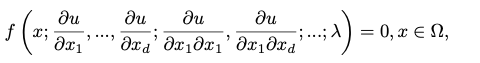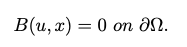# Physics-Informed Neural Networks

Using the PINNs solver, we can solve general nonlinear PDEs:with suitable boundary conditions:where time t is a special component of x, and Ω contains the temporal domain.

PDEs are defined using the ModelingToolkit.jl PDESystem:

@named pde_system = PDESystem(eq,bcs,domains,param,var)

Here, eq is the equation, bcs represents the boundary conditions, param is the parameter of the equation (like [x,y]), and var represents variables (like [u]).

The PhysicsInformedNN discretizer is defined as:

discretization = PhysicsInformedNN(chain,
strategy;
init_params = nothing,
phi = nothing,
derivative = nothing,
)

Keyword arguments:

• chain is a Flux.jl chain, where the input of NN equals the number of dimensions and output equals the number of equations in the system,
• strategy determines which training strategy will be used,
• init_params is the initial parameter of the neural network. If nothing then automatically generated from the neural network,
• phi is a trial solution,
• derivative is a method that calculates the derivative.

The method discretize interprets from the ModelingToolkit PDE form to the PINNs Problem.

prob = discretize(pde_system, discretization)

which outputs an OptimizationProblem for GalacticOptim.jl.

## Training strategy

List of training strategies that are available now:

• GridTraining(dx): Initialize points on a lattice uniformly spaced via dx. If dx is a scalar, then dx corresponds to the spacing in each direction. If dx is a vector, then it should be sized to match the number of dimensions and corresponds to the spacing per direction.

• StochasticTraining(points:bcs_points = ponits): points is number of stochastically sampled points from the domain, bcs_points is number of points for boundary conditions(by default, it equals points). In each optimization iteration, we randomly select a new subset of points from a full training set.

• QuasiRandomTraining(points;bcs_points = ponits, sampling_alg = UniformSample(), resampling = true, minibatch=500): The training set is generated on quasi-random low discrepency sequences. points is the number of quasi-random points in every subset or set, bcs_points is number of points for boundary conditions(by default, it equals points), sampling_alg is the quasi-Monte Carlo sampling algorithm. if resampling = false, the full training set is generated in advance before training, and at each iteration, one subset is randomly selected out of the batch.minibatch is the number of subsets in full training set. The number of the total points is length(lb) * points * minibatch, where lb is the lower bound and length(lb) is the dimensionality. if resampling = true, the training set isn't generated beforehand, and one set of quasi-random points is generated directly at each iteration in runtime. In this case minibatch has no effect.

See the QuasiMonteCarlo.jl for the full set of quasi-random sampling algorithms which are available.

• QuadratureTraining(;quadrature_alg=CubatureJLh(),reltol= 1e-6,abstol= 1e-3,maxiters=1e3,batch=100):

The loss is computed as an approximation of the integral of the PDE loss at each iteration using adaptive quadrature methods via the differentiable Quadrature.jl.

• quadrature_alg is quadrature algorithm,
• reltol: relative tolerance,
• abstol: absolute tolerance,
• maxiters: the maximum number of iterations in quadrature algorithm,
• batch: the preferred number of points to batch. If batch = 0, the number of points in the batch is determined adaptively by the algorithm.

Transfer learning is a machine learning technique where a model trained on one task is re-purposed on a second related task.

• neural_adapter(loss_function,initθ,pde_system,strategy): the method that trains a neural network using the results from one already obtained prediction. Keyword arguments:

• loss_function:the body of loss function,
• initθ: the initial parameter of new neural networks,
• pde_system: PDE are defined using the ModelingToolkit.jl ,
• strategy: determines which training strategy will be used,
• neural_adapter(loss_functions::Array,initθ,pde_systems::Array,strategy): the method that trains a neural network using the results from many already obtained predictions. Keyword arguments:

• loss_functions: the body of loss functions,
• initθ: the initial parameter of the neural network,
• pde_systems: PDEs are defined using the ModelingToolkit.jl,
• strategy: determines which training strategy will be used.

## Low-level API

These additional methods exist to help with introspection:

• symbolic_discretize(pde_system,discretization): This method is the same as discretize but instead returns the unevaluated Julia function to allow the user to see the generated training code.

• build_symbolic_loss_function(eqs,indvars,depvars, phi, derivative, initθ; bc_indvars=nothing): return symbolic inner representation for the loss function. Keyword arguments:

• eqs: equation or equations,
• indvars: independent variables (the parameter of the equation),
• depvars: dependent variables,
• phi:trial solution,
• derivative: method that calculates the derivative,
• initθ: the initial parameter of the neural network,
• bc_indvars: independent variables for each boundary conditions.
• build_symbolic_equation(eq,indvars,depvars): return symbolic inner representation for the equation.

• build_loss_function(eqs, indvars, depvars, phi, derivative, initθ; bc_indvars=nothing): returns the body of loss function, which is the executable Julia function, for the main equation or boundary condition.

• get_loss_function(loss_functions, train_sets, strategy::TrainingStrategies; τ = nothing): return the executable loss function. Keyword arguments:

• loss_functions: the body of loss function, which is created using build_loss_function,
• train_sets: training sets,
• strategy: training strategy,
• τ: normalizing coefficient for loss function. If τ is nothing, then it is automatically set to 1/n where n is the number of points checked in the loss function.
• get_phi(chain): return function for trial solution.

• get_numeric_derivative(): return method that calculates the derivative.

• generate_training_sets(domains,dx,bcs,_indvars::Array,_depvars::Array): return training sets for equations and boundary condition, that is used for GridTraining strategy.

• get_variables(eqs,_indvars::Array,_depvars::Array): returns all variables that are used in each equations or boundary condition.

• get_argument(eqs,_indvars::Array,_depvars::Array): returns all arguments that are used in each equations or boundary condition.

• get_bounds(domains,bcs,_indvars::Array,_depvars::Array): return pairs with lower and upper bounds for all domains. It is used for all non-grid training strategy: StochasticTraining, QuasiRandomTraining, QuadratureTraining.

See how this can be used in Debugging section or 2-D Burgers equation, low-level API examples.